Main Content

# image

Display image from array

## Syntax

``image(C)``
``image(x,y,C)``
``image('CData',C)``
``image('XData',x,'YData',y,'CData',C)``
``image(___,Name,Value)``
``image(ax,___)``
``im = image(___)``

## Description

example

````image(C)` displays the data in array `C` as an image. Each element of `C` specifies the color for 1 pixel of the image. The resulting image is an `m`-by-`n` grid of pixels where `m` is the number of rows and `n` is the number of columns in `C`. The row and column indices of the elements determine the centers of the corresponding pixels. ```

example

````image(x,y,C)` specifies the image location. Use `x` and `y` to specify the locations of the corners corresponding to `C(1,1)` and `C(m,n)`. To specify both corners, set `x` and `y` as two-element vectors. To specify the first corner and let `image` determine the other, set `x` and `y` as scalar values. The image is stretched and oriented as applicable.```
````image('CData',C)` adds the image to the current axes without replacing existing plots. This syntax is the low-level version of `image(C)`. For more information, see High-Level Versus Low-Level Version of Image.```
````image('XData',x,'YData',y,'CData',C)` specifies the image location. This syntax is the low-level version of `image(x,y,C)`.```

example

````image(___,Name,Value)` specifies image properties using one or more name-value pair arguments. You can specify image properties with any of the input argument combinations in the previous syntaxes.```
````image(ax,___)` creates the image in the axes specified by `ax` instead of in the current axes (`gca`). The option `ax` can precede any of the input argument combinations in the previous syntaxes.```

example

````im = image(___)` returns the `Image` object created. Use `im` to set properties of the image after it is created. You can specify this output with any of the input argument combinations in the previous syntaxes. For a list of image properties and descriptions, see Image Properties.```

## Examples

collapse all

Create matrix `C`. Display an image of the data in `C`. Add a colorbar to the graph to show the current colormap.

```C = [0 2 4 6; 8 10 12 14; 16 18 20 22]; image(C) colorbar```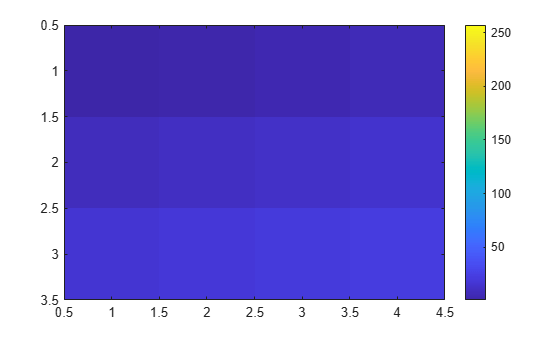By default, the `CDataMapping` property for the image is set to `'direct'` so `image` interprets values in `C` as indices into the colormap. For example, the bottom right pixel corresponding to the last element in `C`, 22, uses the 22nd color of the colormap.

Scale the values to the full range of the current colormap by setting the `CDataMapping` property to `'scaled'` when creating the image.

```image(C,'CDataMapping','scaled') colorbar```Alternatively, you can use the `imagesc` function to scale the values instead of using `image(C,'CDataMapping','scaled')`. For example, use `imagesc(C)`.

Place the image so that it lies between 5 and 8 on the x-axis and between 3 and 6 on the y-axis.

```x = [5 8]; y = [3 6]; C = [0 2 4 6; 8 10 12 14; 16 18 20 22]; image(x,y,C)```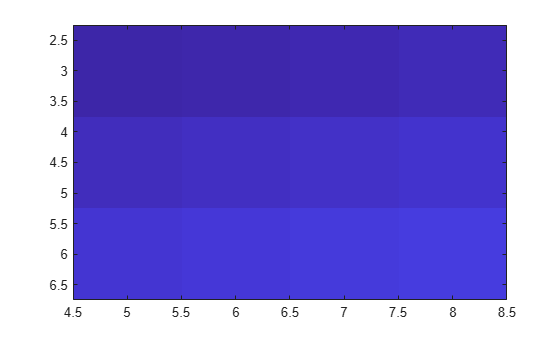Notice that the pixel corresponding to C(1,1) is centered over the point (5,3). The pixel corresponding to C(3,4) is centered over the point (8,6). `image` positions and orients the rest of the image between those two points.

Create `C` as a 3-D array of true colors. Use only red colors by setting the last two pages of the array to zeros.

```C = zeros(3,3,3); C(:,:,1) = [.1 .2 .3; .4 .5 .6; .7 .8 .9]```
```C = C(:,:,1) = 0.1000 0.2000 0.3000 0.4000 0.5000 0.6000 0.7000 0.8000 0.9000 C(:,:,2) = 0 0 0 0 0 0 0 0 0 C(:,:,3) = 0 0 0 0 0 0 0 0 0 ```

Display an image of the data in `C`.

`image(C)`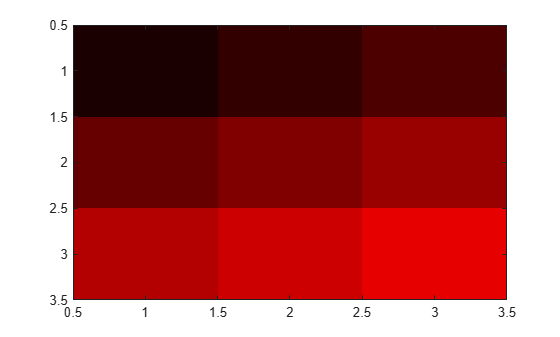Plot a line, and then create an image on top of the line. Return the image object.

```plot(1:3) hold on C = [1 2 3; 4 5 6; 7 8 9]; im = image(C);```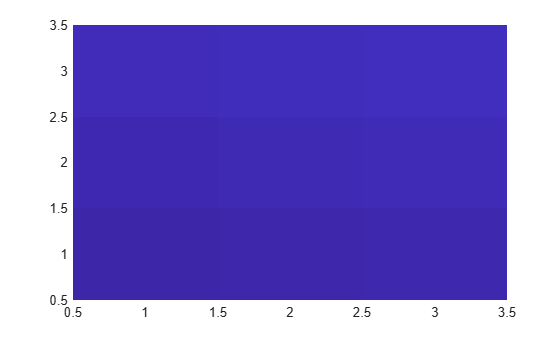Make the image semitransparent so that the line shows through the image.

`im.AlphaData = 0.5;`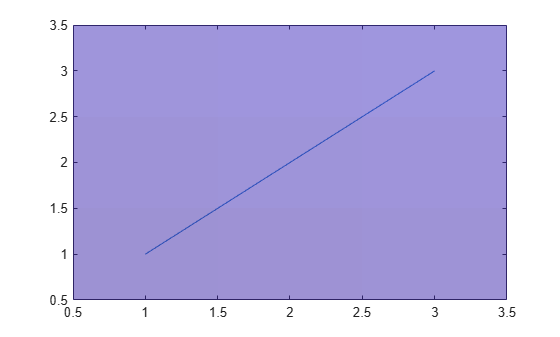Read a JPEG image file.

`C = imread('ngc6543a.jpg');`

`imread` returns a 650-by-600-by-3 array, `C`.

Display the image.

`image(C)`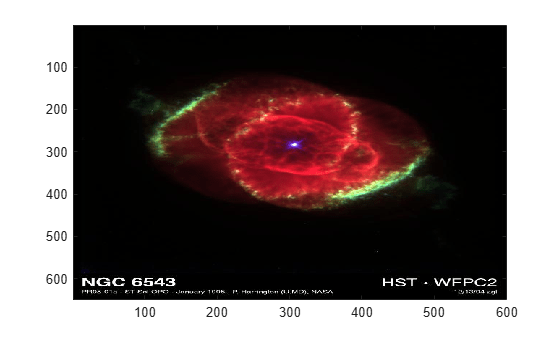Create a surface plot. Then, add an image under the surface. `image` displays the image in the xy-plane.

```Z = 10 + peaks; surf(Z) hold on image(Z,'CDataMapping','scaled')```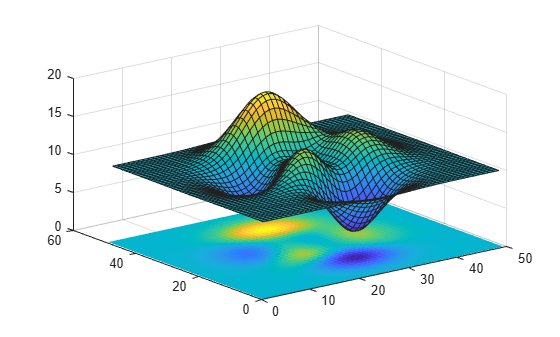## Input Arguments

collapse all

Image color data, specified in one of these forms:

• Vector or matrix — This format defines indexed image data. Each element of `C` defines a color for 1 pixel of the image. For example, ```C = [1 2 3; 4 5 6; 7 8 9];```. The elements of `C` map to colors in the colormap of the associated axes. The `CDataMapping` property controls the mapping method.

• 3-D array of RGB triplets — This format defines true color image data using RGB triplet values. Each RGB triplet defines a color for 1 pixel of the image. An RGB triplet is a three-element vector that specifies the intensities of the red, green, and blue components of the color. The first page of the 3-D array contains the red components, the second page contains the green components, and the third page contains the blue components. Since the image uses true colors instead of colormap colors, the `CDataMapping` property has no effect.

• If `C` is of type `double`, then an RGB triplet value of `[0 0 0]` corresponds to black and `[1 1 1]` corresponds to white.

• If `C` is an integer type, then the image uses the full range of data to determine the color. For example, if `C` is of type `uint8`, then ```[0 0 0]``` corresponds to black and `[255 255 255]` corresponds to white. If `CData` is of type `int8`, then `[-128 -128 -128]` corresponds to black and ```[127 127 127]``` corresponds to white.

• If `C` is of type `logical`, then `[0 0 0]` corresponds to black and ```[1 1 1]``` corresponds to white.

This illustration shows the relative dimensions of `C` for the two color models.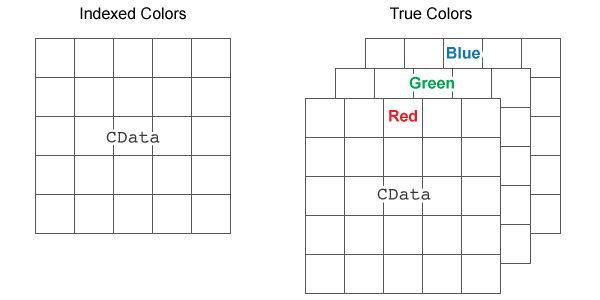The behavior of `NaN` elements is not defined.

To use the low-level version of the `image` function instead, set the `CData` property as a name-value pair. For example, `image('CData',C)`.

#### Converting Between `double` and Integer Data Types

When you call the `image` function with a vector or 2-D matrix and use the default `CDataMapping` value, you must offset your data values by 1 when converting between `double` values and integer types. This offset is not necessary when `CDataMapping` is set to `'scaled'`.

For example, if `U8` contains indexed image data of type `uint8`, you can convert it to type `double` using:

`D = double(U8) + 1;`

To convert indexed image data from type `double` to an integer type, subtract 1 and use `round` to ensure that all the values are integers. For example, if `D` contains indexed image data of type `double`, convert it to `uint8` using:

`U8 = uint8(round(D - 1));`

#### Converting Between Normalized `double` and Truecolor Values

To convert true color image data from an integer type to type `double`, rescale the data. For example, if `RGB8` is true color image data of type `uint8`, convert it to `double` using:

`RGB = double(RGB8)/255;`

To convert true color image data from type `double` to an integer type, rescale the data and use `round` to ensure that all the values are integers. For example, if `RGB` is image data of type `double`, convert it to `uint8` using:

`RGB8 = uint8(round(RGB*255));`

Data Types: `single` | `double` | `int8` | `int16` | `int32` | `int64` | `uint8` | `uint16` | `uint32` | `uint64` | `logical`

Placement along the x-axis, specified in one of these forms:

• Two-element vector — Use the first element as the location for the center of `C(1,1)` and the second element as the location for the center of `C(m,n)`, where `[m,n] = size(C)`. If `C` is a 3-D array, then `m` and `n` are the first two dimensions. Evenly distribute the centers of the remaining elements of `C` between those two points.

The width of each pixel is determined by the expression:

`(x(2)-x(1))/(size(C,2)-1)`

If `x(1)` > `x(2)`, then the image is flipped left-right.

• Scalar — Center `C(1,1)` at this location and each following element one unit apart.

To use the low-level version of the `image` function instead, set the `XData` property as a name-value pair. For example, `image('XData',x,'YData',y,'CData',C)`.

You cannot interactively pan or zoom outside the x-axis limits or y-axis limits of an image, unless the limits are already set outside the bounds of the image. If the limits are already outside the bounds, there is no such restriction. If other objects (such as a line) occupy the axes and extend beyond the bounds of the image, you can pan or zoom to the bounds of the other objects, but no further.

Data Types: `single` | `double` | `int8` | `int16` | `int32` | `int64` | `uint8` | `uint16` | `uint32` | `uint64` | `logical`

Placement along y-axis, specified in one of these forms:

• Two-element vector — Use the first element as the location for the center of `C(1,1)` and the second element as the location for the center of `C(m,n)`, where `[m,n] = size(C)`. If `C` is a 3-D array, then `m` and `n` are the first two dimensions. Evenly distribute the centers of the remaining elements of `C` between those two points.

The height of each pixel is determined by the expression:

`(y(2)-y(1))/(size(C,1)-1)`

If `y(1)` > `y(2)`, then the image is flipped up-down.

• Scalar — Center `C(1,1)` at this location and each following element one unit apart.

To use the low-level version of the `image` function instead, set the `YData` property as a name-value pair. For example, `image('XData',x,'YData',y,'CData',C)`.

You cannot interactively pan or zoom outside the x-axis limits or y-axis limits of an image, unless the limits are already set outside the bounds of the image. If the limits are already outside the bounds, there is no such restriction. If other objects (such as a line) occupy the axes and extend beyond the bounds of the image, you can pan or zoom to the bounds of the other objects, but no further.

Data Types: `single` | `double` | `int8` | `int16` | `int32` | `int64` | `uint8` | `uint16` | `uint32` | `uint64` | `logical`

`Axes` object. If you do not specify an `Axes` object, then `image` uses the current axes.

### Name-Value Pair Arguments

Specify optional comma-separated pairs of `Name,Value` arguments. `Name` is the argument name and `Value` is the corresponding value. `Name` must appear inside quotes. You can specify several name and value pair arguments in any order as `Name1,Value1,...,NameN,ValueN`.

Example: `image([1 2 3],'AlphaData',0.5)` displays a semitransparent image.

The properties listed here are a subset of image properties. For a complete list, see Image Properties.

Color data mapping method, specified as `'direct'` or `'scaled'`. Use this property to control the mapping of color data values in `CData` into the colormap. `CData` must be a vector or a matrix defining indexed colors. This property has no effect if `CData` is a 3-D array defining true colors.

The methods have these effects:

• `'direct'` — Interpret the values as indices into the current colormap. Values with a decimal portion are fixed to the nearest lower integer.

• If the values are of type `double` or `single`, then values of `1` or less map to the first color in the colormap. Values equal to or greater than the length of the colormap map to the last color in the colormap.

• If the values are of type `uint8`, `uint16`, `uint32`, `uint64` , `int8`, `int16`, `int32`, or `int64`, then values of `0` or less map to the first color in the colormap. Values equal to or greater than the length of the colormap map to the last color in the colormap (or up to the range limits of the type).

• If the values are of type `logical`, then values of `0` map to the first color in the colormap and values of `1` map to the second color in the colormap.

• `'scaled'` — Scale the values to range between the minimum and maximum color limits. The `CLim` property of the axes contains the color limits.

Transparency data, specified in one of these forms:

• Scalar — Use a consistent transparency across the entire image.

• Array the same size as `CData` — Use a different transparency value for each image element.

The `AlphaDataMapping` property controls how MATLAB® interprets the alpha data transparency values.

Example: `0.5`

Data Types: `single` | `double` | `int8` | `int16` | `int32` | `int64` | `uint8` | `uint16` | `uint32` | `uint64` | `logical`

Interpretation of `AlphaData` values, specified as one of these values:

• `'none'` — Interpret the values as transparency values. A value of 1 or greater is completely opaque, a value of 0 or less is completely transparent, and a value between 0 and 1 is semitransparent.

• `'scaled'` — Map the values into the figure’s alphamap. The minimum and maximum alpha limits of the axes determine the alpha data values that map to the first and last elements in the alphamap, respectively. For example, if the alpha limits are `[3 5]`, then alpha data values less than or equal to `3` map to the first element in the alphamap. Alpha data values greater than or equal to `5` map to the last element in the alphamap. The `ALim` property of the axes contains the alpha limits. The `Alphamap` property of the figure contains the alphamap.

• `'direct'` — Interpret the values as indices into the figure’s alphamap. Values with a decimal portion are fixed to the nearest lower integer:

• If the values are of type `double` or `single`, then values of 1 or less map to the first element in the alphamap. Values equal to or greater than the length of the alphamap map to the last element in the alphamap.

• If the values are of type integer, then values of 0 or less map to the first element in the alphamap. Values equal to or greater than the length of the alphamap map to the last element in the alphamap (or up to the range limits of the type). The integer types are `uint8`, `uint16`, `uint32`, `uint64` , `int8`, `int16`, `int32`, and `int64`.

• If the values are of type `logical`, then values of 0 map to the first element in the alphamap and values of 1 map to the second element in the alphamap.

## Output Arguments

collapse all

`Image` object, returned as a scalar. Use `im` to set properties of the image after it is created. For a list, see Image Properties.

## More About

collapse all

### High-Level Versus Low-Level Version of Image

The `image` function has two versions, the high-level version and the low-level version. If you use `image` with `'CData'` as an input argument, then you are using the low-level version. Otherwise, you are using the high-level version.

The high-level version of `image` calls `newplot` before plotting and sets these axes properties:

• `Layer` to `'top'`. The image is shown in front of any tick marks or grid lines.

• `YDir` to `'reverse'`. Values along the y-axis increase from top to bottom. To decrease the values from top to bottom, set `YDir` to `'normal'`. This setting reverses both the y-axis and the image.

• `View` to `[0 90]`.

The low-level version of the `image` function does not call `newplot` and does not set these axes properties.

## Tips

• To read image data into MATLAB from graphics files in various standard formats, such as TIFF, use `imread`. To write MATLAB image data to graphics files, use `imwrite`. The `imread` and `imwrite` functions support a variety of graphics file formats and compression schemes.

## See Also

### Properties

Introduced before R2006a

Download ebook